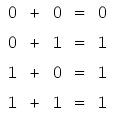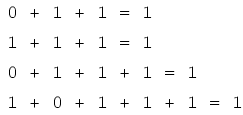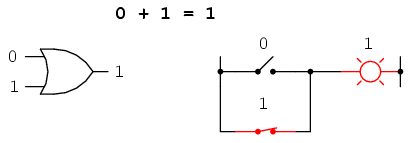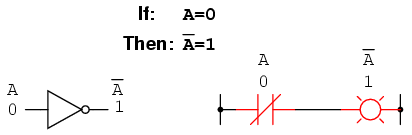# 7.2: Boolean Arithmetic

Let us begin our exploration of Boolean algebra by adding numbers together:The first three sums make perfect sense to anyone familiar with elementary addition. The last sum, though, is quite possibly responsible for more confusion than any other single statement in digital electronics, because it seems to run contrary to the basic principles of mathematics. Well, it does contradict principles of addition for real numbers, but not for Boolean numbers. Remember that in the world of Boolean algebra, there are only two possible values for any quantity and for any arithmetic operation: 1 or 0. There is no such thing as “2” within the scope of Boolean values. Since the sum “1 + 1” certainly isn’t 0, it must be 1 by process of elimination.

It does not matter how many or few terms we add together, either. Consider the following sums:Take a close look at the two-term sums in the first set of equations. Does that pattern look familiar to you? It should! It is the same pattern of 1’s and 0’s as seen in the truth table for an OR gate. In other words, Boolean addition corresponds to the logical function of an “OR” gate, as well as to parallel switch contacts:There is no such thing as subtraction in the realm of Boolean mathematics. Subtraction implies the existence of negative numbers: 5 - 3 is the same thing as 5 + (-3), and in Boolean algebra negative quantities are forbidden. There is no such thing as division in Boolean mathematics, either, since division is really nothing more than compounded subtraction, in the same way that multiplication is compounded addition.

Multiplication is valid in Boolean algebra, and thankfully it is the same as in real-number algebra: anything multiplied by 0 is 0, and anything multiplied by 1 remains unchanged:This set of equations should also look familiar to you: it is the same pattern found in the truth table for an AND gate. In other words, Boolean multiplication corresponds to the logical function of an “AND” gate, as well as to series switch contacts:Like “normal” algebra, Boolean algebra uses alphabetical letters to denote variables. Unlike “normal” algebra, though, Boolean variables are always CAPITAL letters, never lower-case. Because they are allowed to possess only one of two possible values, either 1 or 0, each and every variable has a complement: the opposite of its value. For example, if variable “A” has a value of 0, then the complement of A has a value of 1. Boolean notation uses a bar above the variable character to denote complementation, like this:In written form, the complement of “A” denoted as “A-not” or “A-bar”. Sometimes a “prime” symbol is used to represent complementation. For example, A’ would be the complement of A, much the same as using a prime symbol to denote differentiation in calculus rather than the fractional notation d/dt. Usually, though, the “bar” symbol finds more widespread use than the “prime” symbol, for reasons that will become more apparent later in this chapter.

Boolean complementation finds equivalency in the form of the NOT gate, or a normally-closed switch or relay contact:The basic definition of Boolean quantities has led to the simple rules of addition and multiplication, and has excluded both subtraction and division as valid arithmetic operations. We have a symbology for denoting Boolean variables, and their complements. In the next section we will proceed to develop Boolean identities.

REVIEW:

• Boolean addition is equivalent to the OR logic function, as well as parallel switch contacts.
• Boolean multiplication is equivalent to the AND logic function, as well as series switch contacts.
• Boolean complementation is equivalent to the NOT logic function, as well as normally-closed relay contacts.